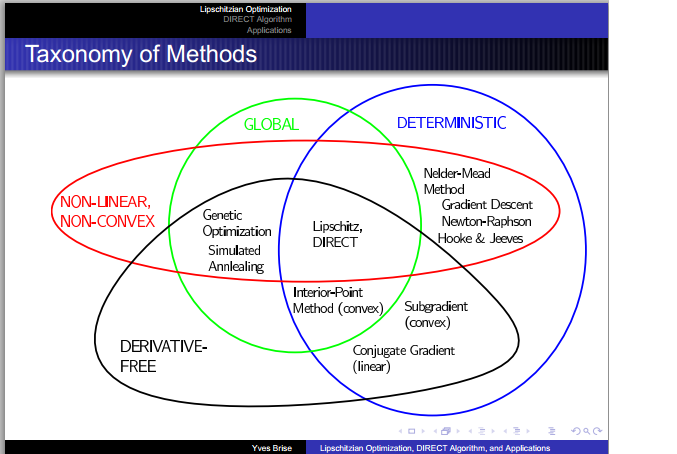# Matlab code and a Tutorial on DIRECT OptimizationYves Brise created this nice set of slides describing the DIRECT algorithm for Lipschitz functions.  Tim Kelly of Drexel university provides Matlab code here.

1.The slide shown doesn’t appear right. Nelder-mead, and Hooke-Jeaves are both derivative free.

2.Yes, neither Nelder-Mead nor Hooke-Jeaves use the actual derivative.

Nelder-Mead in effect does a gradient descent. By evaluating the function at the vertices of the simplex, it figures out approximately the direction of the gradient and uses that to determine the next evaluation. So it is quite similar to steepest descent.

Hooke-Jeaves also is similar to gradient descent because it evaluates points near the best current estimate of the minimum.

So despite the fact that they are derivative free, it seems to me that they behave similarly to gradient descent.

3.Also, Conjugate Gradient is not derivative free.